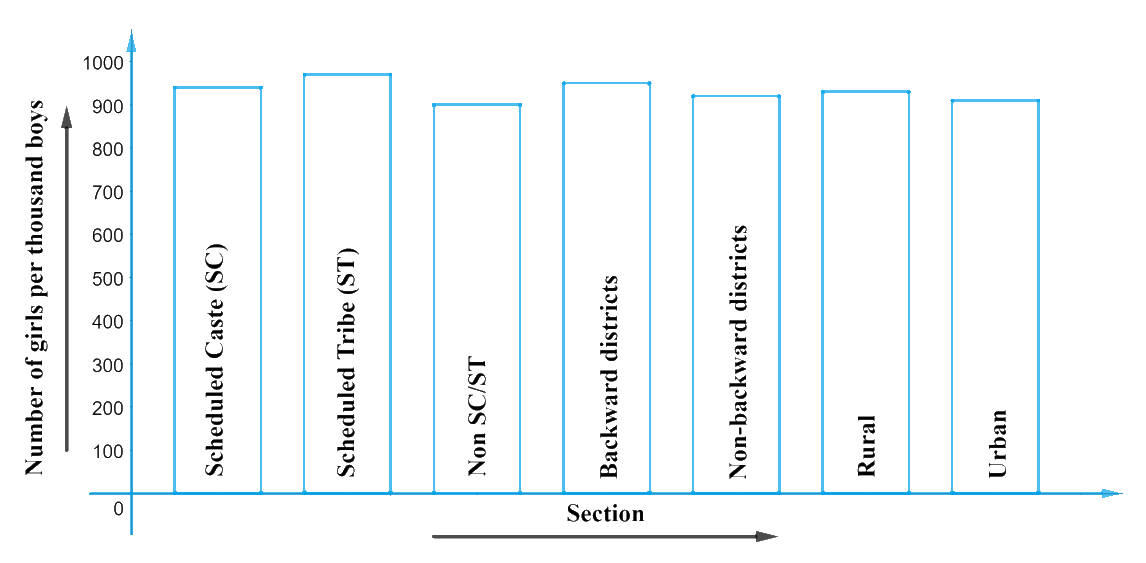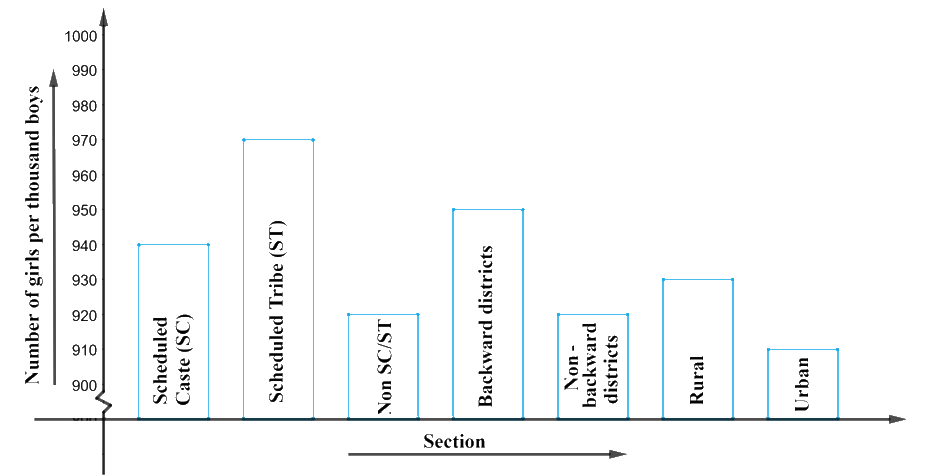# Ex.14.3 Q2 Statistics Solution - NCERT Maths Class 9

Go back to  'Ex.14.3'

## Question

The following data on the number of girls (to the nearest ten) per thousand boys in different sections of Indian society is given below.

 Section Number of girls per thousand boys Scheduled Caste (SC) $$940$$ Scheduled Tribe (ST) $$970$$ Non SC/ST $$920$$ Backward districts $$950$$ Non-backward districts $$920$$ Rural $$930$$ Urban $$910$$

(i) Represent the information above by a bar graph.

(ii) In the classroom discuss what conclusions can be arrived at from the graph.

Video Solution
Statistics
Ex exercise-14-3 | Question 2

## Text Solution

What is known?

The number of girls (to the nearest ten) per thousand boys in different sections of Indian society is given

Unknown:

1. Graphical representation of the given information.
2. Conclusions that can be arrived from the graph.

Reasoning:

The above data can be represented graphically using a bar graph as below:

1. Represent the ‘section’ on the $$x$$-axis and ‘number of girls per thousand boys on the $$y$$-axis.
2. We will select a scale of $$1\,\rm unit = 100$$ girls for $$y$$-axis as the max value did not exceed low.
3. We will maintain equal width for all the bars in $$x$$-axis and also maintain equal gaps in between the bars.

Steps:It can be observed from the graph that:

1. The number of girls per thousand boys is highest for $$ST$$ category.
2. The number of girls per thousand boys is lowest for urban category.
3. The ‘number of given / $$1000$$’ boys are higher in rural area than in urban.
4. The ‘number of given / $$1000$$’ boys are also higher in backward districts them in non-backward districts.

Point to note:

1. The variations in values occur mostly in the range of $$900-970.$$
2. The values are same until $$900.$$
3. Hence to make the variations more visible and distinct and for a better study of the graph, we can keep the starting value on the $$y$$-axis as ‘$$900$$’ with a scale of $$1\,\rm unit$$ as ‘$$10$$’ and re construct the graph as shown below:Number of girls / thousands of boys

As you can see, the above graph shows a clear picture of the data and helps us to arrive at conclusions easily.

Learn from the best math teachers and top your exams

• Live one on one classroom and doubt clearing
• Practice worksheets in and after class for conceptual clarity
• Personalized curriculum to keep up with school